## Complex-Valued Baseband to Real-Valued Passband Up- and Downconversion¶

As OFDM is modulated as a complex-valued baseband signal, we need to perform upconversion to the carrier frequency at the transmitter side to obtain a real-valued transmittable signal. At the receiver side, we need to convert the real-valued received signal to the complex-valued baseband. Here, we roughly follow the description from DSPIllustrations.com on the up- and downconversion.

Let us describe the up- and downconversion as follows. We start with complex-valued baseband samples $x[k]$ which are sampled at the baseband bandwidth $B$. These samples are first sent through an interpolation filter $g(t)$. This filter describes the conversion from discrete time (sampled with rate $B$) to continuous time. Mathematically, we can describe the discrete-time baseband $x_B(t)$ signal as follows:

$$x_B(t) = \sum_k x[k]\delta(t-k/B)$$

This signal is then fltered by the interpolation filter $g(t)$ by

$$x_{LP}(t) = x_B(t)*g(t)=\sum_k x[k] g(t-k/B)$$

and the resulting signal is finally upconverted to the carrier frequency $f_c$ by

$$x_{BP}(t) = \text{Re}\{x_{LP}(t)\cdot\exp(j2\pi f_c t)\}$$

to obtain the real-valued transmit signal. At the receiver side, the process is reversed. First, the received bandpass signal $y_{BP}(t)$ is downconverted to baseband

$$y_{Down}(t)=y_{BP}(t)\cdot \exp(-j2\pi f_c t)$$

to obtain a complex-valued signal. This is signal is subsequently lowpass filtered with the matched filter to $g(t)$. Assuming $g(t)$ is symmetric, $g(t)$ is its own matched filter, and hence

$$y_{LP}(t)=g(t)*y_{Down}(t).$$

Finally, the lowpass-filtered signal is sampled at rate $B$ to obtain the desired baseband samples

$$y[k] = y_{LP}(k/B).$$

If we would like to implement this procedure, we face a fundamental problem: How can we control the continuous-time signal? We only have access to the discrete-time samples that we will send to the soundcard! The actual discrete-time to continuous-time conversion is performed by the soundcard itself where we have no control of the filter etc.!

Here, we have no option but to accept this limitation. However, we can still use the above described upconversion procedure: Let us assume, we use small bandwidths $B$ for the baseband (e.g. $B=441\mathrm{Hz}$). In relation to $B$ the sampling rate $F$ of the soundcard of $F=44100\mathrm{Hz}$ is already close to continuous-time. Hence, we just consider the sampling rate $F$ of the sound card as the continuous-time scale, and the samples we work with are the low-rate $B$ baseband samples.

Hence, in our implementation in addtion to upconversion, we need to perform upsampling from $B$ to $F$ at the transmitter side. At the receiver, in addition to downconversion, we need to do the decimation.

Let us now write the according components for up- and downconversion. However, first, let's write a simple signal-generator component to test the chain:

In :
# Source code has been redacted in online version
# Omitting 4 lines of source code

In :
class TransmitSignal(TX):
def __init__(self, environment, B, generateSignalFunc):
super().__init__(environment)
self._B = B             # the bandwidth of the baseband signal (i.e. baseband samplerate)
self._generateSignalFunc = generateSignalFunc

self._numsamples = 0    # the number of up to now transmitted samples

def _generateSignal(self):
N = 2048
t = (np.arange(N) + self._numsamples) / self._B
self._numsamples += N
return self._generateSignalFunc(t)


Let us try this component:

In :
# Source code has been redacted in online version
# Omitting 11 lines of source code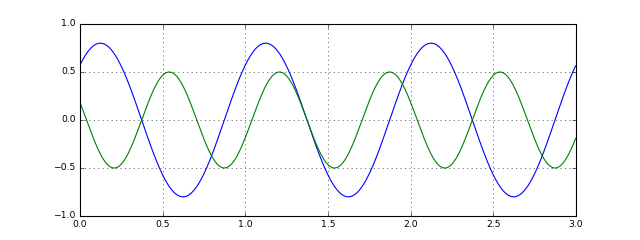OK, the component works and emits a complex signal given by the passed lambda function.

Now, let's proceed and implement the upconversion, interpolation and downconversion and decimation components: First, we define the filter $g$ that is used for interpolation. Here, we use a root-Nyquist filter, such that after the matched filtering at the receiver side, no ISI occurs.

In :
# The filter used for the up- and downsampling of the baseband signal
def UpDownConversionFilter(B, samplerate):
N = 3
t = np.arange(-N/B, N/B, 1/samplerate)
rrc_samples = get_filter('rrc', 1/B, rolloff=0.15)(t) * np.sqrt(B / samplerate)

return StatefulFilter(rrc_samples, np.array())


Now we are ready to implement the upconversion. The implementation is straight-forwardly performing the operations

\begin{align} x_B(t) &= \sum_k x[k]\delta(t-k/B) \\ x_{LP}(t) &= x_B(t)*g(t)=\sum_k x[k] g(t-k/B) \\ x_{BP}(t) &= \text{Re}\{x_{LP}(t)\cdot\exp(j2\pi f_c t)\} \end{align}
In :
# Source code has been redacted in online version
# Omitting 27 lines of source code


Let us now look at the output signal of the upconversion. This time, we transmit a simple sine in the baseband:

In :
# Source code has been redacted in online version
# Omitting 18 lines of source code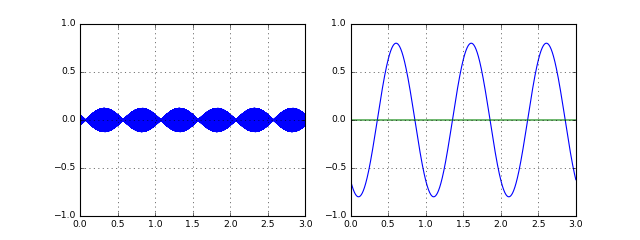Stop received from external


Yes, the channel output looks reasonable. Let us now proceed to the downconversion unit at the receiver side. The receiver unit simply applies the following operations to the received signal $y_BP(t)$:

\begin{align} y_{Down}(t)&=y_{BP}(t)\cdot \exp(-j2\pi f_c t) \\ y_{LP}(t)&=g(t)*y_{Down}(t) \\ y[k] &= y_{LP}(k/B). \end{align}

The only difficulty arises from the fact that the number of incoming samples is not necessarily a multiple of the interpolation factor, hence we need to keep track of the sample count at the receiver to support uniform decimation.

In :
class DownconversionDecimation(Component):
def __init__(self, environment, Fc, B):
super().__init__(environment, None)
self._Fc = Fc
self._B = B

assert self._samplerate % B == 0, "The samplerate must be a multiple of the baseband bandwidth!"
self._U = self._samplerate // B  # The decimation rate
self._LPfilter = UpDownConversionFilter(B, self._samplerate)

self._numsamples = 0  # Number of processed incoming samples
self._nextoff = 0     # Number of samples from the next incoming block to be ignored as part of the decimation

if len(data) == 0:
return

# keep track of already processed sample count and increase time accordingly
t = (np.arange(len(data)) + self._numsamples) / self._samplerate
self._numsamples += len(data)

# Downconversion
e = np.exp(-2j*np.pi*self._Fc*t)
y_down = data * e

y_LP = self._LPfilter.filter(y_down)

# Decimation
yk = np.sqrt(2)*y_LP[self._nextoff::self._U]

remaining = (len(y_LP)-self._nextoff) % self._U
self._nextoff = (self._U - remaining) % self._U

if len(yk) > 0:
self._send(yk)


OK, with this straight-forward implementation, let us see if the passband transmission works. We write the following generic function for our tests:

In :
def runTransmission(channelFunc, generateSignalFunc, duration=1, deltaF=0):
env = Environment(samplerate=44100)

B = 441
Fc = 2000

transmitter = TransmitSignal(environment=env, B=B, generateSignalFunc=generateSignalFunc)
upc = InterpolationUpconversion(env, Fc=Fc, B=B)
channel = channelFunc(env)
downc = DownconversionDecimation(env, Fc=Fc+deltaF, B=B)
fig = env.figure(figsize=(10,3))
d = 0.4
showTXBaseband = PlotWaveform(environment=env, windowDuration=d, rate=B, ylim=(-1,1), isComplex=True, axes=fig.add_subplot(131), title='TX base band')
showPassband = PlotWaveform(environment=env, windowDuration=d, ylim=(-0.5, 0.5), axes=fig.add_subplot(132), title='Passband')
showRXBaseband = PlotWaveform(environment=env, windowDuration=d, rate=B, ylim=(-1,1), isComplex=True, axes=fig.add_subplot(133), title='RX base band')
rec = Recorder(environment=env, duration=duration)

transmitter.transmitsTo(upc)
transmitter.transmitsTo(showTXBaseband)
upc.transmitsTo(channel)
channel.transmitsTo(downc)
channel.transmitsTo(rec)
channel.transmitsTo(showPassband)
downc.transmitsTo(showRXBaseband)

env.run()


Let us look at the up- and downconversion output for an ideal channel:

In :
runTransmission(SimulatedChannel, lambda t: 0.8*np.sin(2*np.pi*10*t)+0.5j*np.cos(2*np.pi*15*t))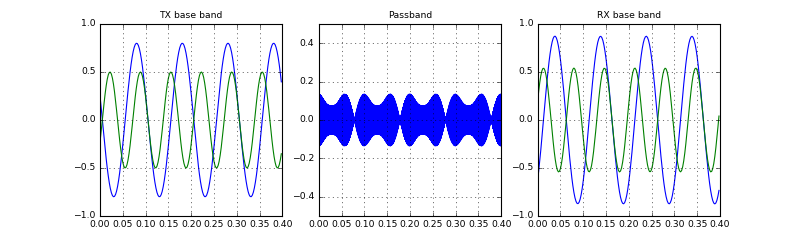Cool! Despite a time-shift, the received signal looks equal to transmitted baseband signal. Now, let's look at what happens when we use the real channel:

In :
runTransmission(AudioChannel, lambda t: 0.8*np.sin(2*np.pi*10*t)+0.5j*np.cos(2*np.pi*15*t), duration=5)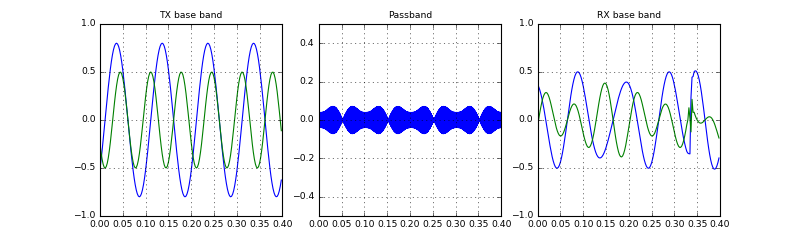Hm, with the real audio channel, the received baseband signal is different from the transmitted. Let's analyze this a bit further by transmitting a simpler signal, i.e. a constant signal:

In :
runTransmission(AudioChannel, lambda t: 0*t+0.8, duration=5)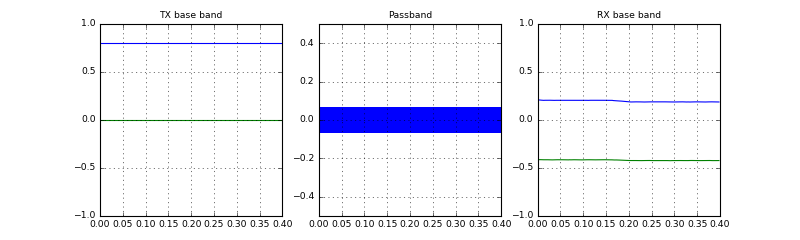Hm... it seems that the audio channel is incorporating a phase rotation: We transmit a real-valued constant signal, and receive a constant, but complex-valued signal. (It might also happen, that the received signal even changes over time. This is due to some buffer underrun or other impairments).

How can we explain or model this behaviour? We know that at the receiver side, a decimation operation is performed:

$$y[k] = y_{LP}(k/B)$$

Here, we sample the lowpass-filtered signal with a rate $B$. However, at the receiver side we do not know, at which exact time instances the sampling should happen. To do this, the receiver would need to exactly know the delay between the transmitter and the receiver. This is impossible, especially when a random multipath channel with variable delay is between the transmitter and receiver. Let's see, if a different delay between transmitter and receiver creates the same effect. To introduce a delay we write a simple channel effect that throws away the first few samples:

In :
class InitialLossChannelEffect(SimulatedChannelEffect):
def __init__(self, numLostSamples):
super().__init__()
self._numLostSamples = numLostSamples

def response(self, signal):
if self._numLostSamples > len(signal):
self._numLostSamples -= len(signal)
return
elif self._numLostSamples > 0:
result = signal[self._numLostSamples:]
self._numLostSamples = 0
return result
else:
return signal

In :
# Source code has been redacted in online version
# Omitting 6 lines of source code


### No lost samples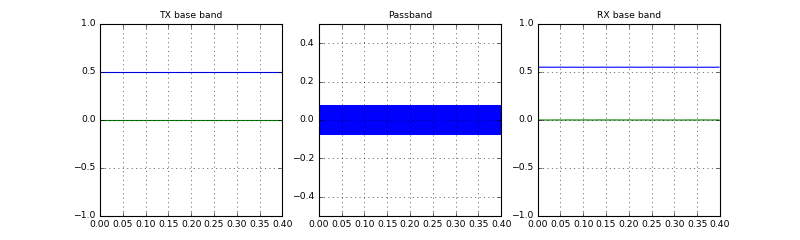### 1 lost sample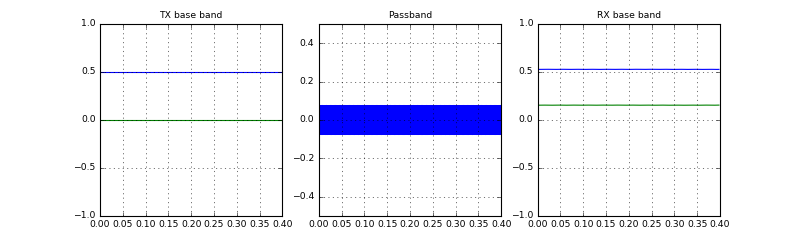### 10 lost samples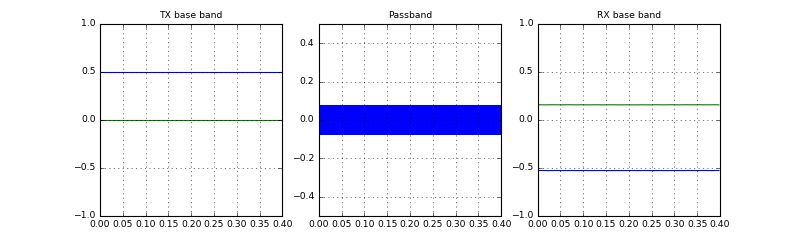Ah, so losing some samples (i.e. introducing extra delay) changes the phase of the output signal. This is exactly the behaviour we observed with the audio channel. Let us go one step further and use a channel that constantly loses samples on a random basis:

In :
class LoseSamplesChannelEffect(SimulatedChannelEffect):
def response(self, signal):
rate = 0.0001
keep = np.random.rand(len(signal)) > rate
return signal[keep]

In :
runTransmission(lambda env: SimulatedChannel(env, channelEffect=LoseSamplesChannelEffect()), lambda t: t*0+0.5, duration=10)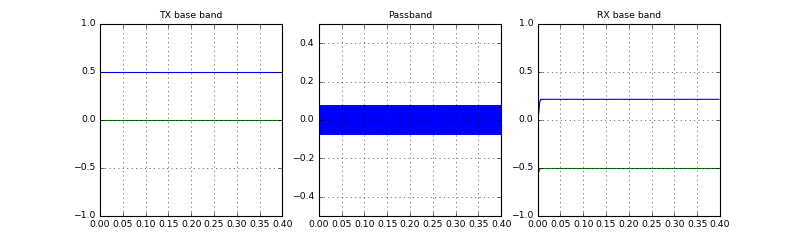Ah, constantly losing samples and hence constantly increasing the delay between transmitter and receiver a continuous phase shift of the base band signal. Let's finally look again at the signal from the audio channel:

In :
runTransmission(AudioChannel, lambda t: t*0+0.5, duration=10)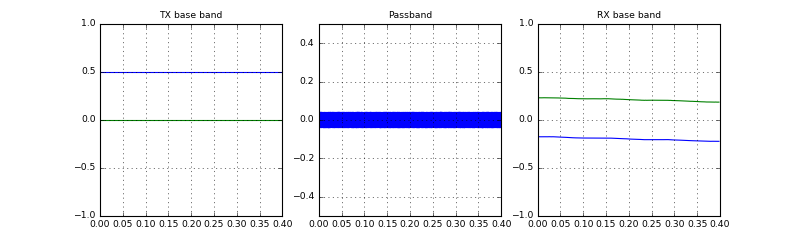Stop received from external


OK, as we saw before, the audio channel creates a random phase shift between TX and RX baseband signal. Hence, an important aspect at the receiver side is to estimate this phase shift as part of the channel estimation block.

### Summary¶

In this notebook, we have set up the up- and downconversion such that we can model our systems in complex-valued baseband and transmit it over the real audio channel. We have seen that the downconversion procedure introduces a complex phase shift depending on the delay between transmitter and receiver and hence needs to be estimated and compensated at the receiver side.

In the next notebook, we will shortly talk about the design of the AD/DA conversion filter. Afterwards, we will start working with OFDM by looking at fundamental OFDM modulation and demodulation.